Tutorials For Excel everyday with EVBA.info part 1 - EBOOK VBA EXCELEVBA.info ( Ebook VBA Excel ) Welcome EVBA.info - Nothing Is Unable ... About Excel Tricks, Learning VBA Programming, Dedicated Software, Free Courses, Living Skills ...

## Friday, October 25, 2019

When you put hyperlinks in cells on a worksheet, it’s a good practice to present them in a user-friendly, intuitive way. For example, the pictures show an original list of hyperlinked cells with their Microsoft-induced wordy screen tips. The modified list was created by the below macro to show those same cells more intuitively, with displayed text and screen tips that are concise and easy to read.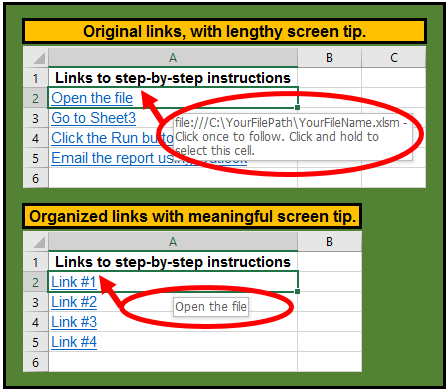`Sub EditLinks()Dim myHype As HyperlinkDim iLink As Integer, strText As StringiLink = 1For Each myHype In Columns(1).HyperlinksstrText = myHype.Parent.TextmyHype.TextToDisplay = "Link #" & iLinkmyHype.ScreenTip = strTextiLink = iLink + 1Next myHypeEnd Sub`
Tom’s Tutorials For Excel: Conditionally Formatting Early, Late, and Acceptable Times
Here is how you can use Conditional Formatting to identify times that are more than, or less than, a margin of acceptability. In the picture, the cells holding airline flight arrival times that occur more than 60 seconds earlier than their scheduled arrival time are shaded yellow. The Conditional Formatting formula in cell B12 copied down to cell B22 for the yellow cells is
`=B12 < A12-(1/1440)`.
Cells holding an actual arrival time more than 1 minute later than their scheduled time are shaded blue. Their Conditional Formatting formula in cell B12 copied down to cell B22 for the blue cells is
`=B12 > A12+(1/1440)`.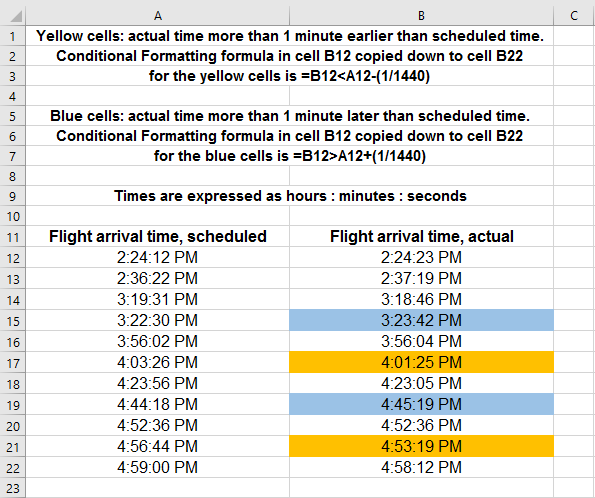Tom’s Tutorials For Excel: Analyzing Named Ranges with the INDIRECT Function
The INDIRECT function can refer to a named range for quick data analysis, especially if you don’t need or want to use a pivot table. In this example, columns B:F hold several years of daily sales activity for a department store. The next picture shows a list of named ranges in the name box that correspond to the header labels.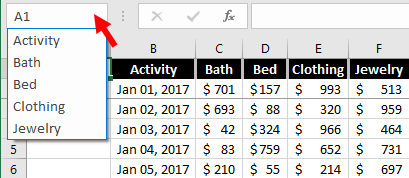The cells in range H2:J2 are data validated. Suppose you want to see historical data for Clothing sales in February 2015. In the next picture, year 2015 is selected in cell H2.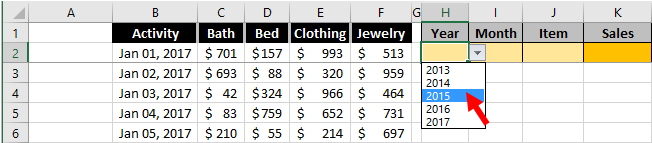Next, month 2 is selected, for February in cell I2.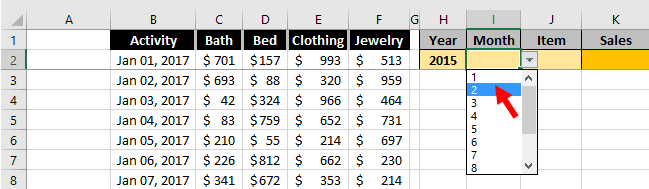Finally, the Clothing item is selected in cell J2.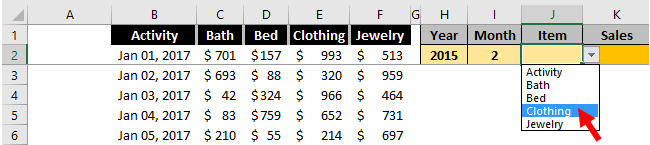This formula in cell K2 returns Sales of \$13,756:
`=SUMPRODUCT((YEAR(Activity)=H2)+0,(MONTH(Activity)=I2)+0,INDIRECT(J2))`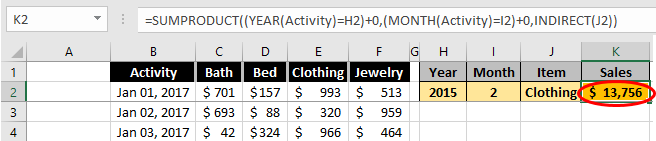Verifying the formula, here is the range for Clothing sales in February 2015.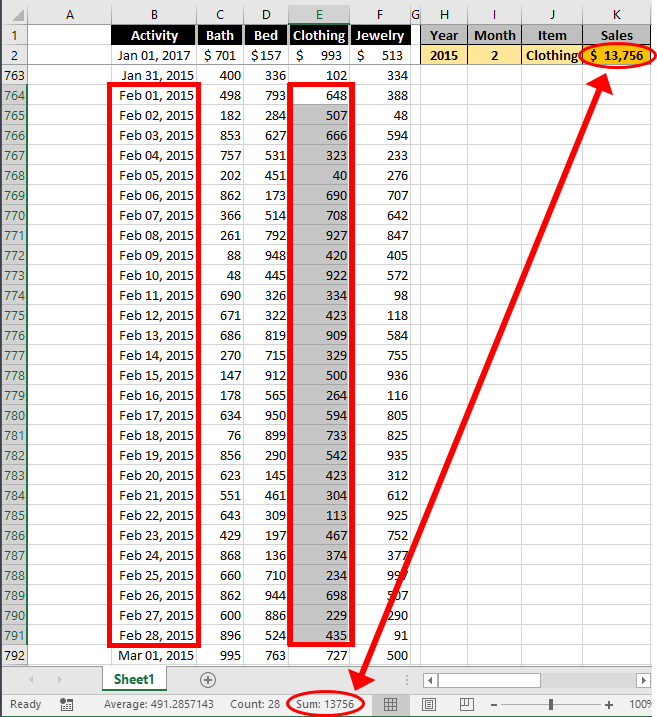Tom’s Tutorials For Excel: Using Undo For Several Actions at Once
You can Undo up to 100 previous actions on your workbook at the same time. Clicking the drop-down arrow next to the Undo button shows the list of your most recent actions, where you can select the point at which to undo the action(s) you want to reverse. The Redo button, next to the Undo button, restores what you reverse.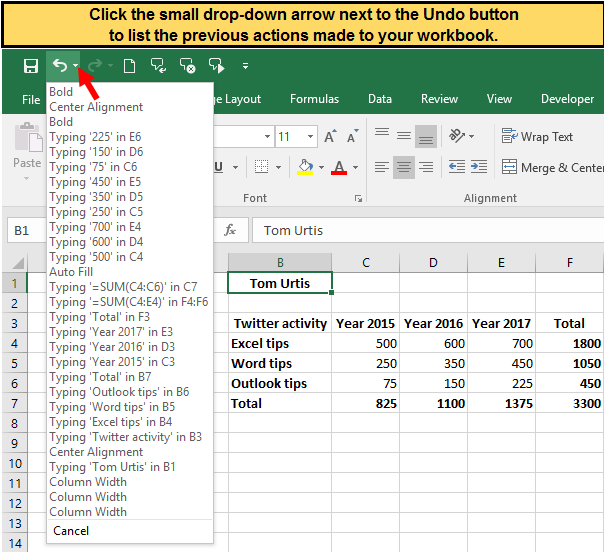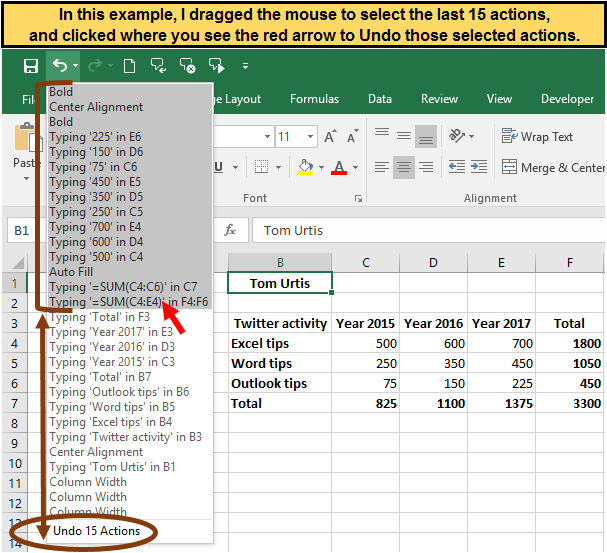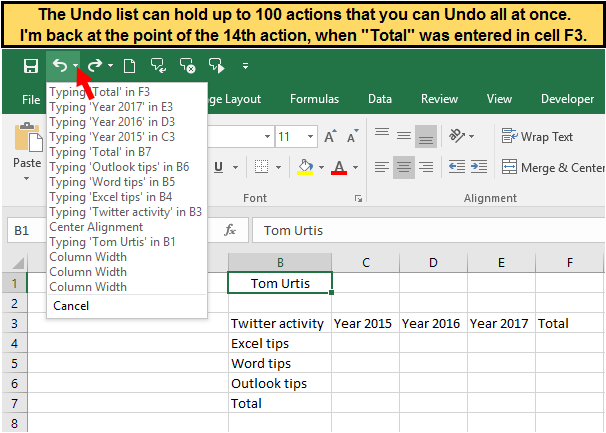Tom’s Tutorials For Excel: Bypassing the Start screen when opening Excel
When you open Excel, here is how to bypass the Start screen when you prefer to see a new workbook instead.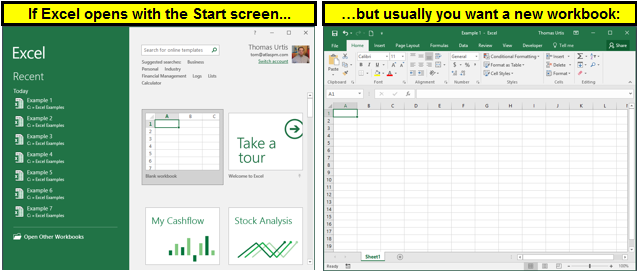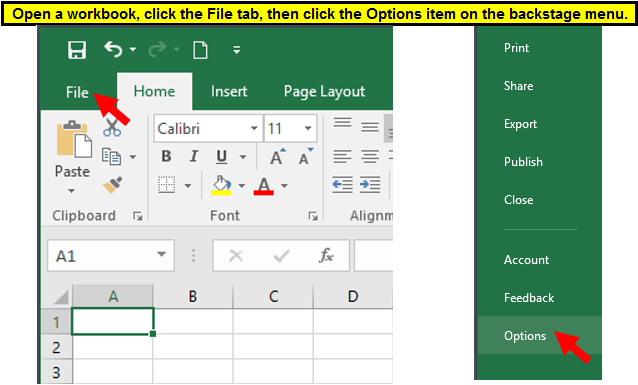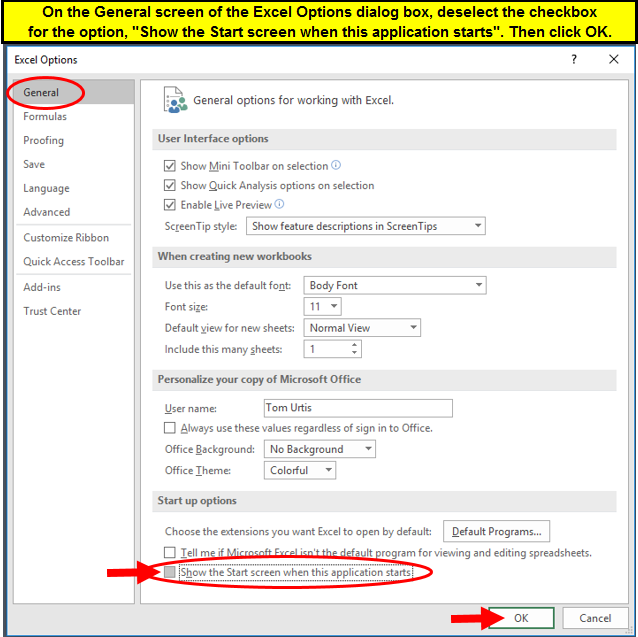Tom’s Tutorials For Excel: Using Label Headers as Intersecting Lookup Criteria
I previously posted this example of using the spacebar character as the mathematical operator in a formula to sum numbers in the intersecting range of multiple rows and columns. Also is this other example of using a formula to lookup an intersecting value. Here is a combination of those two methods, using the location of row and column header labels to return their intersecting cell’s value. In the first picture, a worksheet holds data for each region’s quarterly numbers in range B2:F6. Yellow cells H3 and I3 are data validated for lists of Quarter labels in column A, and Region labels in row 1.
The green cells hold the lookup result.
The formula in cell H8 is
`=IF(LEN(I8)=0,"","Lookup result:")`
The formula in cell I8 is
`=IFERROR(INDIRECT(ADDRESS(MATCH(H3,A:A,0),MATCH(I3,1:1,0))),"")`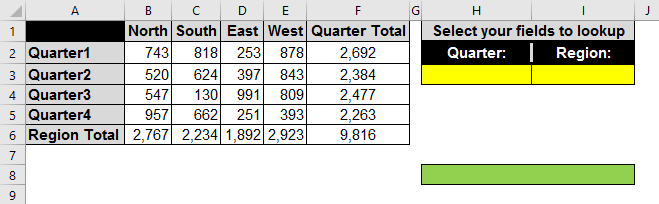In the next picture, you select your Quarter field name.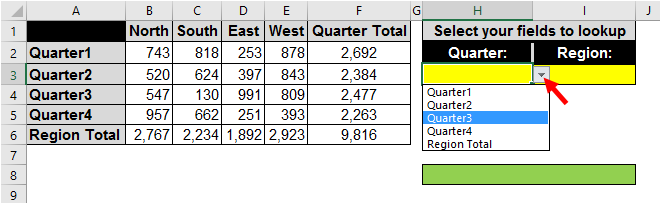In the next picture, you select your Region field name.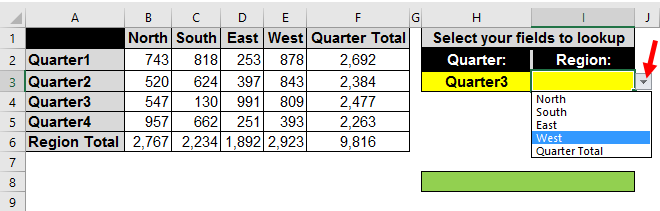Here is the resulting value at the intersection of those 2 selected criteria.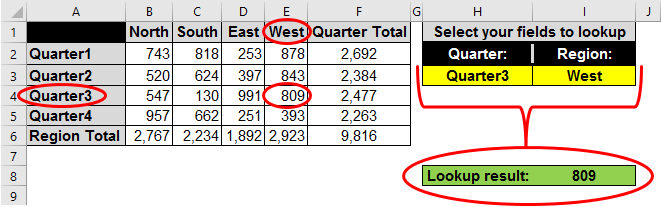Tom’s Tutorials For Excel: Copying Only a PivotTable’s PivotField to Another Worksheet
Here is how you can copy a specific pivot field and display its data on another worksheet. In the following pictures, only the columns of a pivot table’s Sales field are copied to Sheet2 so you can work with that Sales data independent of the original pivot table. The `CopyPivotField` macro’s code purposely includes the State headers and the Grand Total rows in this example with the `Offset` and `Resize` methods, which you can modify if you don’t want those rows in your copied data.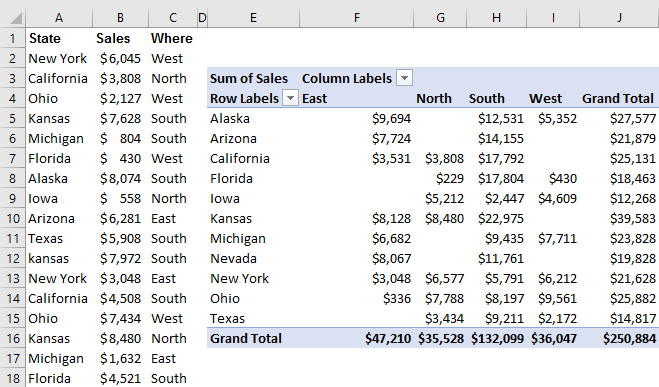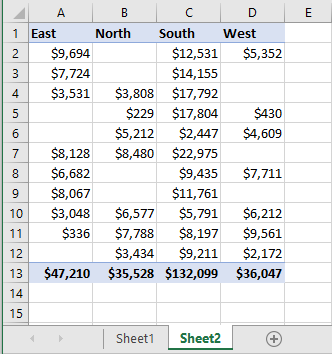`Sub CopyPivotField()With ActiveSheet.PivotTables(1)With .PivotFields("Sum of Sales").DataRange.Offset(-1).Resize(.Rows.Count + 2).Copy Sheet2.Range("A1")End WithEnd WithEnd Sub`
This one-liner (the line continuation underscore character is for readability on the web page) in a macro or in the Immediate window copies a PivotField’s data without a header or totals row:
`ActiveSheet.PivotTables(1).PivotFields("Sum of Sales") _.DataRange.Copy Sheet2.Range("A1")`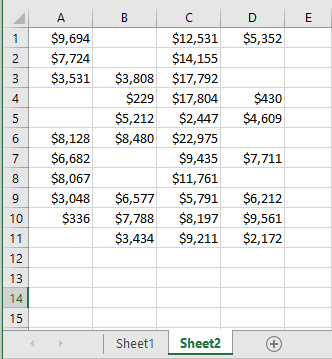## Tom’s Tutorials For Excel: Reverse Lookup For Times in Milliseconds

Tom’s Tutorials For Excel: Reverse Lookup For Times in Milliseconds
You can format, analyze, and lookup (in this example, reverse VLOOKUP with CHOOSE) times measured in milliseconds.
In the first picture, times for a swimming race are measured in minutes, seconds, and milliseconds. The times in range B4:B12, and in cell B14, are custom format `m:ss:000` as shown in the second picture.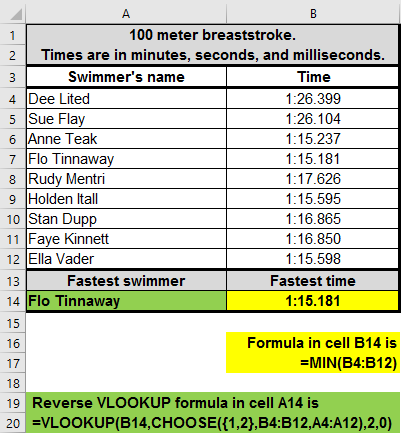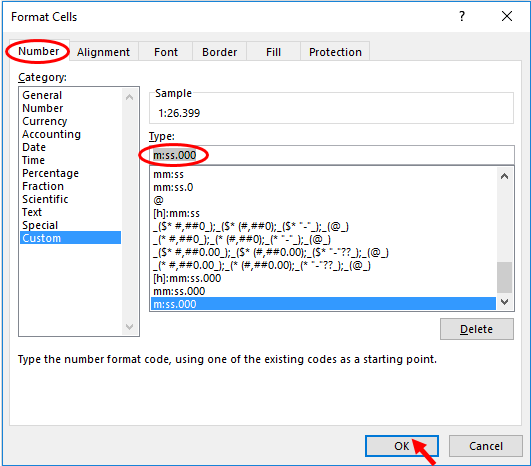Tom’s Tutorials For Excel: Opening Excel in a New Instance
In Excel versions 2013 and later, new workbooks open in the same instance of Excel. Here are 2 options to open your workbook in its own instance.
Option 1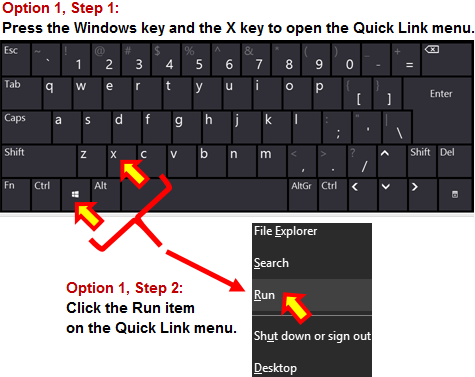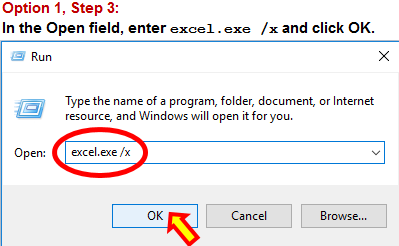Option 2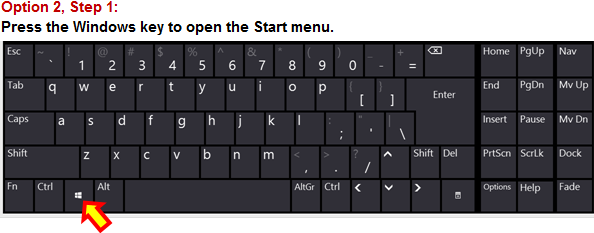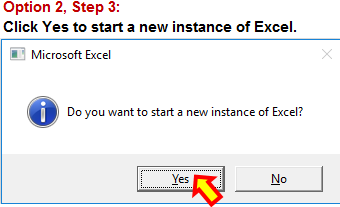Tom’s Tutorials For Excel: Averaging Positive and Negative Numbers With Multiple Criteria
When crunching numbers, there are many ways to slice and dice the Average onion, depending on what criteria you want to include, exclude, combine, or isolate.
The picture shows a list of positive and negative numbers, and an analysis calculation table with 12 formula examples of varying multiple criteria. Notice the subtle syntax differences in formulas between the `AVERAGEIF` function, and the `AVERAGE` function with nested `IF` and `ABS` arguments.
Some of these formulas are arrays, which I’ve noted below. An array formula is applied to a cell by simultaneously pressing the `Ctrl+Shift+Enter` keys, not just `Enter`. The curly braces are not typed by you; Excel places them automatically when the array formula is properly applied. If you are unfamiliar with array formulas, see my video and explanation of arrays here.
Calculating “Average yardage result of all plays”:
The formula in cell F13 is `=AVERAGE(C5:C24)`
Calculating “Average yardage change all plays (as if all positive)”:
The array formula in cell F14 is `=AVERAGE(ABS(C5:C24))`
Calculating “Average of positive plays (only for yards gained)”:
The formula in cell F15 is `=AVERAGEIF(C5:C24,">0")`
Calculating “Average of negative plays (only for yards lost)”:
The formula in cell F16 is `=AVERAGEIF(C5:C24,"<0")`
Calculating "Average of all Run plays only":
The formula in cell F17 is `=AVERAGEIF(B5:B24,"=Run",C5:C24)`
Calculating "Average of all Pass plays only":
The formula in cell F18 is `=AVERAGEIF(B5:B24,"=Pass",C5:C24)`
Calculating "Average Run yardage change (as if all positive)":
The array formula in cell F19 is `=AVERAGE(IF(B5:B24="Run",ABS(C5:C24)))`
Calculating "Average Pass yardage change (as if all positive)":
The array formula in cell F20 is `=AVERAGE(IF(B5:B24="Pass",ABS(C5:C24)))`
Calculating "Average of positive Run plays (that gained yards)":
The array formula in cell F21 is `=AVERAGE(IF(B5:B24="Run",IF(C5:C24>0,C5:C24)))`
Calculating "Average of negative Run plays (that lost yards)":
The array formula in cell F22 is `=AVERAGE(IF(B5:B24="Run",IF(C5:C24<0,C5:C24)))`
Calculating "Average of positive Pass plays (that gained yards)":
The array formula in cell F23 is `=AVERAGE(IF(B5:B24="Pass",IF(C5:C24>0,C5:C24)))`
Calculating "Average of negative Pass plays (that lost yards)":
The array formula in cell F24 is `=AVERAGE(IF(B5:B24="Pass",IF(C5:C24<0,C5:C24)))`
📤You download App EVBA.info installed directly on the latest phone here : https://www.evba.info/p/app-evbainfo-setting-for-your-phone.html?m=1